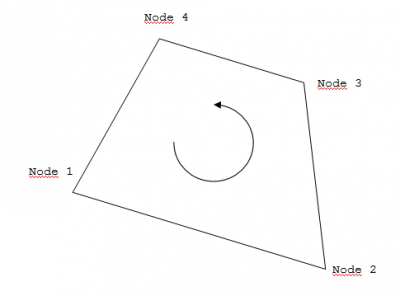# NEMOH-Mesh

#### Mesh format

The mesh is composed of flat quadrangular panels. Only the submerged part of the body must be described.

The format of a mesh file is:
 2 1 First number must be a 2. Seconde number is 1 if a symmetry about the (xOz) place is used. 0 otherwise 1 5. 0. - 10. Table of nodes. First number is the node ID. Other numbers are x y and z coordinates . . . . 0 0. 0. 0. Last line of table of nodes 1 2 3 4 Table of connectivities. Numbers of node IDs. . . . . 0 0 0 0 Last line of table of connectivities
The sketch below shows the orientation convention used to describe the panel as seen looking from the fluid domain into the body.It is possible to consider several bodies with different degrees of freedom with hydrodynamic interactions. This is obtained by meshing separetely each body. The assembled mesh will be defined in the Nemoh.cal input file and built by the preProcessor.

#### Mesh generation

There are several alternatives to generate meshes for NEMOH. Some tools are provided in order to help. However, one may use any CAD software provided that one will be able to export the mesh to the correct format for NEMOH.

#### Mesh generation using the Matlab routines axiMesh.m or Mesh.m

One may use axiMesh.m to generate an axisymmetric mesh with Matlab. Only the line generating the profile needs to be described in this case. The axis will be necessarily vertical in this case. As an example, here is how to generate a vertical cylinder with 10m diameter and 5m draft:

>> n=4;
>> r=[0 5 5 0];
>> z=[5 5 -5 -5];
>> [Mass,Inertia,KH,XB,YB,ZB]=axiMesh(r,z,n)

Non axisymmetric meshes may be generated using Mesh.m. However it is assumed that a symmetry about the (xOz) plane is used, thus only half of the body must be described. This routine also allows to generate meshes with several interacting bodies. In this case one will have to describe roughly the geometries. Mesh will be refined automatically and part above sea water level will be cut out. As an example, here is how to generate a mesh for a regular box with 10m length and 5m draft:

>> nBodies=1;
>> n=5;
>> X(1,1,:,:)=[-5. -5. 5.;-5 -5. -5.;5. -5. -5.;5. -5. 5.];
>> X(1,2,:,:)=[-5. 0. 5.;-5 -5. 5.;5. -5. 5.;5. 0. 5.];
>> X(1,3,:,:)=[-5. 0. -5.;5 0. -5.;5. -5. -5.;-5. -5. -5.];
>> X(1,4,:,:)=[5. 0. 5.;5 -5. 5.;5. -5. -5.;5. 0. -5.];
>> X(1,5,:,:)=[-5. 0. 5.;-5 0. -5.;-5. -5. -5.;-5. -5. 5.];
>> tX(1)=0.;
>> CG(1,:)=[0. 0. -2.5];
>> nfobj(1)=300;
>> [Mass,Inertia,KH,XB,YB,ZB]=Mesh(nBodies,n,X,tX,CG,nfobj)

#### Mesh generation using the Mesh code

The Mesh code is not a meshing software. Actually, it allows to refine a mesh and to calculate properties such as displacement, buoyancy center, hydrostatic stiffness. It also makes estimate of masses and inertia matrix. The concept with this software is to write by hand a coarse description of the body under consideration and to have Mesh make the refined mesh for NEMOH calculations.

Input files are:
• ID.dat file: identification file, as for NEMOH.
• Mesh file: this file contains the (coarse) description of the body. It is normally made by hand. The format is a little different than for NEMOH:
 100 Number of nodes 25 Number of panels 5. 0. -10. Table of nodes. Numbers are the x and z coordinates . . . 1 2 3 3 Table of connectivities. Other numbers ar node IDs. . . . .

• Mesh.cal file: input file. The format is:
 test.dat String of character: name of mesh file
 0 1 if a symmetry about (xOz) is used. 0 otherwise 0 0. Possible translation about x axis (first number) and y axis (second number) 0 0. -3. Coordinates of gravity centre 500 Target for the number of panels in refined mesh 2 0 1 1025 Water density (kg/m3) 9.81 Gravity (m/s2)
Output files are:

• /Mesh/Hydrostatics.dat file: this file contains the coordinates of the buoyancy center, the displacement and the waterplane area.

• /Mesh/KH.dat file: hydrostatic stiffness matrix of the body.

• /Mesh/Description_Full.tec and /Mesh/Description_Wetted.tec: These files are in a Tecplot compatible format. They may be used for vizualisation of the input description of the body.

• /Mesh/GC_hull.dat and /Mesh/Inertia_hull.dat files: Coordinates of the gravity centre of the body and inertia matrix. These values are derived using the assumption that the mass of the body is equal to its displacement and that the mass is distributed on the surface of the body.

• /Mesh/mesh_file_name.dat and /Mesh/mesh_file_name.tec file: First file is the mesh to be used with NEMOH. Second file is for vizualisation with Tecplot.

• /Mesh/mesh_file_name_info.dat file: This file contains the information relative to the number of points and panels in the mesh file for Nemoh.